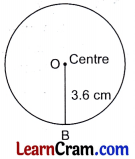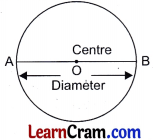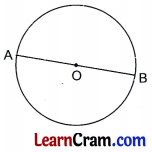# DAV Class 6 Maths Chapter 13 Worksheet 2 Solutions

The DAV Maths Book Class 6 Solutions and DAV Class 6 Maths Chapter 13 Worksheet 2 Solutions of Circles offer comprehensive answers to textbook questions.

## DAV Class 6 Maths Ch 13 WS 2 Solutions

Question 1.
Draw circles whose radius is given below:
(a) 5 cmSteps:
(i) Fix a well sharpened pencil in the compass arm.
(ii) Take a distance of 5 cm between the two arm-points of the compass with the help of scale.
(iii) Mark a point O on your paper sheet and put the the point O.
(iv) Rotate the pencil head round the centre O.
(v) The figure obtained is the required circle with centre O and radius 5 cm.

(b) 4.5 cmSteps:
(i) Fix a well sharpened pencil ¡n the compass-arm.
(ii) Take a distance of 4.5 cm between the two arm-points of the compass with the help of scale.
(iii) Mark a point O on your paper sheet.
(iv) Put the needle arm on the point O.
(v) Rotate the pencil head round the centre 0.
(vi) The figure so obtained is the required circle with centre O and radius 4.5 cm.

(c) 3.6 cmSteps:
(i) Fix a well sharpened pencil in the compass-arm.
(ii) Fix a distance of 3.6 cm between the two arm-points of the compass with the help of a ruler/scale.
(iii) Mark a point O on the paper sheet and put the needle arm on the point O.
(iv) Rotate the pencil head round the centre O.
(v) Hence, the figure so obtained in the required circle with centre O and radius 3.6 cm.

(d) 6.2 cmSteps:
(i) Fix a well sharpened pencil in the compass arm.
(ii) Fix the two arm-points 6.2 cm apart with the help of a A compass.
(iii) Mark a point O on the paper sheet and put the needle arm on the point O.
(iv) Rotate the pencil head round the centre O.
(v) Hence, the figure so obtained is the required circle with centre O and radius 6.2 cm.Question 2.
Draw circles whose diameters are given below. Also draw one radius for each and measure it.
(a) 6 cmSteps:
(i) Fix a well sharpened pencil in the compass arm.
(ii) Take a distance equal to half of the given diameter 6 cm between the two arm-points of the compass with the help of scale.
(iii) Mark a point O on your paper sheet and put the needle arm on the point O.
(iv) Rotate the pencil head round the centre O.
(v) Hence, the figure so obtained is the required circle with centre O and diameter 6 cm.

(b) 8 cmSteps:
(i) Fix a well sharpened pencil in one arm of the compass.
(ii) Measure the distance 4 cm = $$\frac{8}{2}$$cm between the two pointed arms of the compass with the help of a scale.
(iii) Mark a point O on your paper sheet and put the needle arm of the compass at the point O.
(iv) Rotate the pencil head round the centre.
(v) Hence, the figure so obtained is the required circle of diameter AB = 8 cm and centre O

(c) 8.4 cmSteps:
(i) Fix a well sharpened pencil in one arm of the compass.
(ii) Measure the distance 4.2 cm = $$\frac{1}{2}$$ x 8.4 cm between the two pointed arms of the compass with the help of scale.
(iii) Mark a point 0 on your paper- sheet and put the needle arm of the compass at the point O.
(iv) Rotate the pencil head round the centre.
(v) Hence, the figure so obtained is the required circle of diameter PQ = 8.4 cm and centre O.

(d) 5.4 cmSteps;
(i) Fix a small and well sharpened pencil in one arm of the compass.
(ii) Open the two arms of the compass to a distance of 2.7 cm = cm with the help of a scale.
(iii) Mark a point O on your paper sheet and put the needle arm at point O.
(iv) Rotate the pencil head round the point O.
(v) Hence, the figure so obtained is the required circle of diameter AB = 5.4 cm and centre O.Question 3.
Draw a circle of radius 4 cm. Draw its longest chord and measure it.